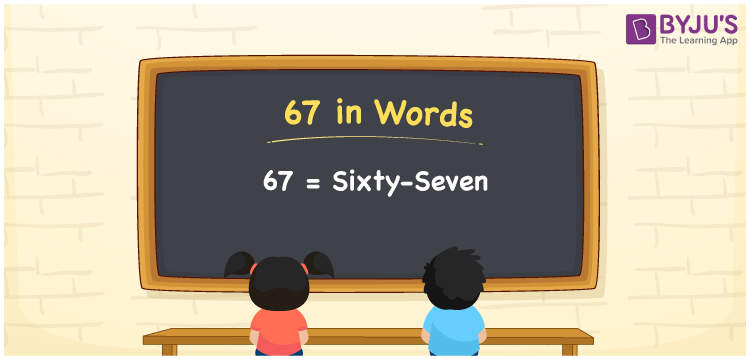# 67 in Words

67 in words is written as “Sixty-seven”. 67 is a two-digit number that has two place values, ones and tens. It represents a count, quantity or value. To represent 67 in words, we should know how to read and write it in English since it is the most commonly used language. Also, learning to spell numbers in words is necessary to utilise in real-life situations. For example, Ravi saved Sixty-seven Rupees. Learn how to write 67 in words in this article.

 67 in words Sixty-seven Sixty-seven in Numbers 67

## 67 in English Words

We generally write numbers in words using the English alphabet. Thus, we can read 67 in English as “Sixty-seven”.## How to Write 67 in Words?

To convert 67 in words, we need to check the place value of its digits. Since 67 is a two-digit number, thus, place value of 6 is tens and 7 is ones. The order of place values of digits in a number is in accordance with the Indian numbering system, such as:

• Ones
• Tens
• Hundreds
• Thousands
• Ten-thousands
• Hundred-thousands or Lakhs

This can be done as follows.

 Tens Ones 6 7

This can be written as:

6 × Ten + 2 × One

= 6 × 10 + 7 × 1

= 60 + 7

= 67

= Sixty-seven

Thus, 67 in words is written as Sixty-seven.

67 is a natural number that precedes 68 and succeeds 66.

67 in words – Sixty-seven

Is 67 an odd number? – Yes

Is 67 an even number? – No

Is 67 a perfect square number? – No

Is 67 a perfect cube number? – No

Is 67 a prime number? – Yes

Is 67 a composite number? – No

## Frequently Asked Questions on 67 in Words

### How do you write 67 in English?

We can write 67 in English words as “Sixty-seven”.

### What is 367 in English?

367 is written as Three hundred and Sixty-seven in English.

### Is 67 an even number or an odd number?

67 is an odd number since it is not completely divisible by 2.